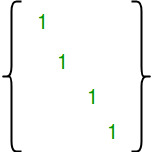# Number of Reflexive Relations on a Set

• Difficulty Level : Easy

Given a number n, find out number of Reflexive Relation on a set of first n natural numbers {1, 2, ..n}.
Examples :

```Input  : n = 2
Output : 4
The given set A = {1, 2}. The following
are reflexive relations on A * A :
{{1, 1), (2, 2)}
{(1, 1), (2, 2), (1, 2)}
{(1, 1), (2, 2), (1, 2), (2, 1)}
{(1, 1), (2, 2), (2, 1)}

Input  : n = 3
Output : 64
The given set is {1, 2, 3}. There are
64 reflexive relations on A * A :```

Explanation :
Reflexive Relation : A Relation R on A a set A is said to be Reflexive if xRx for every element of x ? A.

Attention reader! Don’t stop learning now. Get hold of all the important mathematical concepts for competitive programming with the Essential Maths for CP Course at a student-friendly price. To complete your preparation from learning a language to DS Algo and many more,  please refer Complete Interview Preparation Course.

The number of reflexive relations on an n-element set is 2n2 – n
How does this formula work?
A relation R is reflexive if the matrix diagonal elements are 1.If we take a closer look the matrix, we can notice that the size of matrix is n2. The n diagonal entries are fixed. For remaining n2 – n entries, we have choice to either fill 0 or 1. So there are total 2n2 – n ways of filling the matrix.

## CPP

 `// C++ Program to count reflexive relations``// on a set of first n natural numbers.``#include ``using` `namespace` `std;` `int` `countReflexive(``int` `n)``{``   ``// Return 2^(n*n - n)``   ``return` `(1 << (n*n - n));``}` `int` `main()``{ ``    ``int` `n = 3;``    ``cout << countReflexive(n);``    ``return` `0;``}`

## Java

 `// Java Program to count reflexive``// relations on a set of first n``// natural numbers.` `import` `java.io.*;``import` `java.util.*;` `class` `GFG {``    ` `static` `int` `countReflexive(``int` `n)``{` `// Return 2^(n*n - n)``return` `(``1` `<< (n*n - n));` `}` `// Driver function``    ``public` `static` `void` `main (String[] args) {``    ``int` `n = ``3``;``    ``System.out.println(countReflexive(n));``        ` `    ``}``}` `// This code is contributed by Gitanjali.`

## Python3

 `# Python3 Program to count``# reflexive relations``# on a set of first n``# natural numbers.` ` ` `def` `countReflexive(n):` `    ``# Return 2^(n*n - n)``    ``return` `(``1` `<< (n``*``n ``-` `n));` `# driver function``n ``=` `3``ans ``=` `countReflexive(n);``print` `(ans)` `# This code is contributed by saloni1297`

## C#

 `// C# Program to count reflexive``// relations on a set of first n``// natural numbers.``using` `System;` `class` `GFG {``    ` `    ``static` `int` `countReflexive(``int` `n)``    ``{``    ` `        ``// Return 2^(n*n - n)``        ``return` `(1 << (n*n - n));``        ` `    ``}``    ` `    ``// Driver function``    ``public` `static` `void` `Main () {``        ` `    ``int` `n = 3;``    ``Console.WriteLine(countReflexive(n));``    ``}``}` `// This code is contributed by vt_m.`

## PHP

 ``

## Javascript

 ``

Output :

`64`

My Personal Notes arrow_drop_up